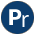#ugraphs.pl -- Graph manipulation library

The S-representation of a graph is a list of (vertex-neighbours) pairs, where the pairs are in standard order (as produced by keysort) and the neighbours of each vertex are also in standard order (as produced by sort). This form is convenient for many calculations.

A new UGraph from raw data can be created using vertices_edges_to_ugraph/3.

Adapted to support some of the functionality of the SICStus ugraphs library by Vitor Santos Costa.

Ported from YAP 5.0.1 to SWI-Prolog by Jan Wielemaker.

author
- R.A.O'Keefe
- Vitor Santos Costa
- Jan Wielemaker
license
- BSD-2 or Artistic 2.0
vertices(+Graph, -Vertices)
Unify Vertices with all vertices appearing in Graph. Example:
```?- vertices([1-[3,5],2-,3-[],4-,5-[]], L).
L = [1, 2, 3, 4, 5]```
vertices_edges_to_ugraph(+Vertices, +Edges, -UGraph) is det
Create a UGraph from Vertices and edges. Given a graph with a set of Vertices and a set of Edges, Graph must unify with the corresponding S-representation. Note that the vertices without edges will appear in Vertices but not in Edges. Moreover, it is sufficient for a vertice to appear in Edges.
```?- vertices_edges_to_ugraph([],[1-3,2-4,4-5,1-5], L).
L = [1-[3,5], 2-, 3-[], 4-, 5-[]]```

In this case all vertices are defined implicitly. The next example shows three unconnected vertices:

```?- vertices_edges_to_ugraph([6,7,8],[1-3,2-4,4-5,1-5], L).
L = [1-[3,5], 2-, 3-[], 4-, 5-[], 6-[], 7-[], 8-[]]```
add_vertices(+Graph, +Vertices, -NewGraph)
Unify NewGraph with a new graph obtained by adding the list of Vertices to Graph. Example:
```?- add_vertices([1-[3,5],2-[]], [0,1,2,9], NG).
NG = [0-[], 1-[3,5], 2-[], 9-[]]```
del_vertices(+Graph, +Vertices, -NewGraph) is det
Unify NewGraph with a new graph obtained by deleting the list of Vertices and all the edges that start from or go to a vertex in Vertices to the Graph. Example:
```?- del_vertices([1-[3,5],2-,3-[],4-,5-[],6-[],7-[2,6],8-[]],
[2,1],
NL).
NL = [3-[],4-,5-[],6-[],7-,8-[]]```
Compatibility
- Upto 5.6.48 the argument order was (+Vertices, +Graph, -NewGraph). Both YAP and SWI-Prolog have changed the argument order for compatibility with recent SICStus as well as consistency with del_edges/3.
add_edges(+Graph, +Edges, -NewGraph)
Unify NewGraph with a new graph obtained by adding the list of Edges to Graph. Example:
```?- add_edges([1-[3,5],2-,3-[],4-,
5-[],6-[],7-[],8-[]],
[1-6,2-3,3-2,5-7,3-2,4-5],
NL).
NL = [1-[3,5,6], 2-[3,4], 3-, 4-,
5-, 6-[], 7-[], 8-[]]```
ugraph_union(+Graph1, +Graph2, -NewGraph)
NewGraph is the union of Graph1 and Graph2. Example:
```?- ugraph_union([1-,2-],[2-,3-[1,2,4]],L).
L = [1-, 2-[3,4], 3-[1,2,4]]```
del_edges(+Graph, +Edges, -NewGraph)
Unify NewGraph with a new graph obtained by removing the list of Edges from Graph. Notice that no vertices are deleted. Example:
```?- del_edges([1-[3,5],2-,3-[],4-,5-[],6-[],7-[],8-[]],
[1-6,2-3,3-2,5-7,3-2,4-5,1-3],
NL).
NL = [1-,2-,3-[],4-[],5-[],6-[],7-[],8-[]]```
graph_subtract(+Set1, +Set2, ?Difference)[private]
Is based on ord_subtract
edges(+Graph, -Edges)
Unify Edges with all edges appearing in Graph. Example:
```?- edges([1-[3,5],2-,3-[],4-,5-[]], L).
L = [1-3, 1-5, 2-4, 4-5]```
transitive_closure(+Graph, -Closure)
Generate the graph Closure as the transitive closure of Graph. Example:
```?- transitive_closure([1-[2,3],2-[4,5],4-],L).
L = [1-[2,3,4,5,6], 2-[4,5,6], 4-]```
transpose_ugraph(Graph, NewGraph) is det
Unify NewGraph with a new graph obtained from Graph by replacing all edges of the form V1-V2 by edges of the form V2-V1. The cost is O(|V|*log(|V|)). Notice that an undirected graph is its own transpose. Example:
```?- transpose([1-[3,5],2-,3-[],4-,
5-[],6-[],7-[],8-[]], NL).
NL = [1-[],2-[],3-,4-,5-[1,4],6-[],7-[],8-[]]```
Compatibility
- This predicate used to be known as transpose/2. Following SICStus 4, we reserve transpose/2 for matrix transposition and renamed ugraph transposition to transpose_ugraph/2.
compose(+LeftGraph, +RightGraph, -NewGraph)
Compose NewGraph by connecting the drains of LeftGraph to the sources of RightGraph. Example:
```?- compose([1-,2-],[2-,3-[1,2,4]],L).
L = [1-, 2-[1,2,4], 3-[]]```
top_sort(+Graph, -Sorted) is semidet
top_sort(+Graph, -Sorted, ?Tail) is semidet
Sorted is a topological sorted list of nodes in Graph. A toplogical sort is possible if the graph is connected and acyclic. In the example we show how topological sorting works for a linear graph:
```?- top_sort([1-, 2-, 3-[]], L).
L = [1, 2, 3]```

The predicate top_sort/3 is a difference list version of top_sort/2.

neighbors(+Vertex, +Graph, -Neigbours) is det
neighbours(+Vertex, +Graph, -Neigbours) is det
Neigbours is a sorted list of the neighbours of Vertex in Graph. Example:
```?- neighbours(4,[1-[3,5],2-,3-[],
4-[1,2,7,5],5-[],6-[],7-[],8-[]], NL).
NL = [1,2,7,5]```
connect_ugraph(+UGraphIn, -Start, -UGraphOut) is det
Adds Start as an additional vertex that is connected to all vertices in UGraphIn. This can be used to create an topological sort for a not connected graph. Start is before any vertex in UGraphIn in the standard order of terms. No vertex in UGraphIn can be a variable.

Can be used to order a not-connected graph as follows:

```top_sort_unconnected(Graph, Vertices) :-
(   top_sort(Graph, Vertices)
->  true
;   connect_ugraph(Graph, Start, Connected),
top_sort(Connected, Ordered0),
Ordered0 = [Start|Vertices]
).```
before(+Term, -Before) is det[private]
Unify Before to a term that comes before Term in the standard order of terms.
Errors
- instantiation_error if Term is unbound.
complement(+UGraphIn, -UGraphOut)
UGraphOut is a ugraph with an edge between all vertices that are not connected in UGraphIn and all edges from UGraphIn removed. Example:
```?- complement([1-[3,5],2-,3-[],
4-[1,2,7,5],5-[],6-[],7-[],8-[]], NL).
NL = [1-[2,4,6,7,8],2-[1,3,5,6,7,8],3-[1,2,4,5,6,7,8],
4-[3,5,6,8],5-[1,2,3,4,6,7,8],6-[1,2,3,4,5,7,8],
7-[1,2,3,4,5,6,8],8-[1,2,3,4,5,6,7]]```
To be done
- Simple two-step algorithm. You could be smarter, I suppose.
reachable(+Vertex, +UGraph, -Vertices)
True when Vertices is an ordered set of vertices reachable in UGraph, including Vertex. Example:
```?- reachable(1,[1-[3,5],2-,3-[],4-,5-[]],V).
V = [1, 3, 5]```

## Undocumented predicates

The following predicates are exported, but not or incorrectly documented.

neighbours(Arg1, Arg2, Arg3)
top_sort(Arg1, Arg2, Arg3)